Create a new printableAnalyze Data and Graphs
Math Worksheets

Sample - Click above to make a new math worksheet (PDF).
 Name _____________________________Date ___________________
Graphs
Complete.
 1 * This is a pre-made sheet.Use the link at the top of the page for a printable page.

Complete.
2.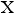3 4 5 6 7 8 9 10 11

How many students read exactly eleven books?

3.1 2 3 4 5 6 7

What is the least number of games won?

Find each ordered pair. Write the letter for the point named by the ordered pair.
4.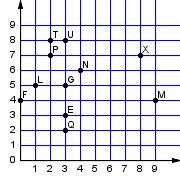(1, 5) _______

 (4, 6) _______

 (0, 4) _______

 (2, 7) _______

 (8, 7) _______
5.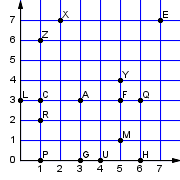(5, 4) _______

 (5, 3) _______

 (1, 3) _______

 (1, 2) _______

 (4, 0) _______

 Key #2
 aKey #2
Complete.
6.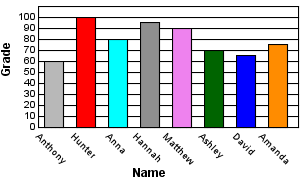a.  Which student(s) had a grade of at least 70?Complete.
7.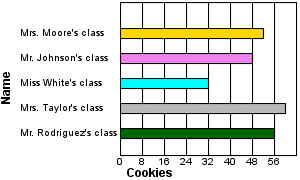a.  How many fewer cookies did Mrs. Moore's class eat than Mrs. Taylor's class?b.  How many classes ate more than 32 cookies?c.  If each student in Mr. Johnson's class ate exactly 4 cookies, how many students are in that class?Complete.
8.
This stem-and-leaf plot shows how long it took each student in a class to complete a homework assignment.
Minutes to Complete Homework
 Stem Leaves 2 7 3 5  7  4  6 4 7  1  8 5 4  9  1 6 3  7  5 7 2  6

 a.  The student who took the longest to complete his or her homework was how much slower than the student who finished his or her homework the fastest?b.  Sort the number of minutes it took students to complete their homework from the slowest time to the quickest time.c.  Is there an outlier? If there is, what is the outlier?Key #2
 aKey #2
Make a vertical bar graph using the data in the table.
9.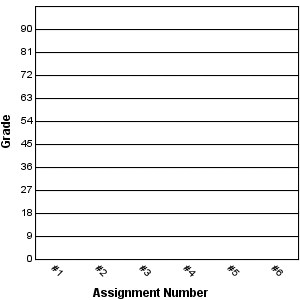Assignment Number Grade #1 75 #2 92 #3 96 #4 97 #5 84 #6 71

 a.  What is the difference between the highest grade and the lowest grade?b.  How many more points were scored on assignment #4 than on assignment #1?c.  Between which two assignments is there a difference of 12 points?Complete.
10.
 Attendance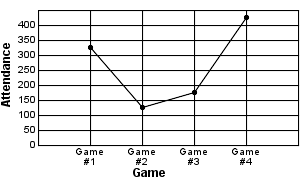a.  Which game had the lowest attendance?b.  How many people attended game #2?c.  Which games(s) had an attendance of more than 325 people?Key #2
 aKey #2
Make a line graph using the data in the table.
11.
 Plant's Height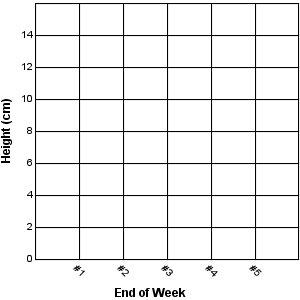Plant's Height
 End of Week Height (cm) #1 3 #2 10 #3 13 #4 14 #5 15

 a.  How much did the plant grow from the end of week #4 to the end of week #5?b.  At the end of which week(s) was the plant no more than 14 centimeters tall?c.  At the end of which week(s) was the plant taller than 14 centimeters?Write the ordered pair for each point.
12.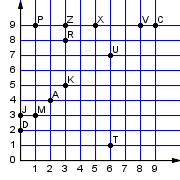R _______

 J _______

 U _______

 M _______

 X _______
13.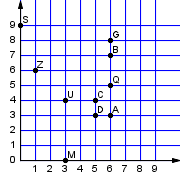D _______

 B _______

 Q _______

 A _______

 Z _______